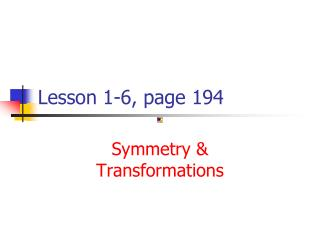DownloadDownload PresentationLesson 1-6, page 194

# Lesson 1-6, page 194

Download Presentation## Lesson 1-6, page 194

- - - - - - - - - - - - - - - - - - - - - - - - - - - E N D - - - - - - - - - - - - - - - - - - - - - - - - - - -
##### Presentation Transcript

1. Lesson 1-6, page 194 Symmetry & Transformations

2. 1.6 Transformation of Functions • Recognize graphs of common functions • Use vertical shifts to graph functions • Use horizontal shifts to graph functions • Use reflections to graph functions • Use vertical stretching & shrinking to graph functions • Use horizontal stretching & shrinking to graph functions • Graph functions w/ sequence of transformations

3. Basic Functions • You should memorize the following basic functions. They are vital to understanding upper level math courses. • See the back section of your book, Algebra’s Common Functions, for all of the basic functions you should memorize. (See inside back cover and turn back 1 or 2 pages.)

4. Types of Transformations • Vertical translation: shifts basic graph up or down; up if y = f(x) + b ordown if y = f(x) – b • Horizontal translation: shifts basic graph left or right; right if y = f(x - d) orleft if y = f(x + d) • Reflection over x-axis: across x axis if y = -[f(x)] • Vertical stretching and shrinking:y = af(x) stretch if |a| > 1; shrink if 0<|a|<1

5. Vertical shifts • Moves the graph up or down • Impacts only the “y” values of the function • No changes are made to the “x” values • Horizontal shifts • Moves the graph left or right • Impacts only the “x” values of the function • No changes are made to the “y” values

6. Recognizing the shift from the equation, look at examples of shifting the function f(x)=x2. • Vertical shift of 3 units up • Horizontal shift of 3 units left (HINT: x’s go the opposite direction of what you might think.)

7. Combining a vertical & horizontal shift • Example of function that is shifted down 4 units and right 6 units from the original function.

8. Types of Symmetry Symmetry with respect to the • y-axis (x, y) & (-x, y) are reflections across the y-axis • Origin (x, y) & (-x, -y) are reflections across the origin • x-axis(x, y) & (x, -y) are reflections across the x-axis

9. Tests of Symmetry • f(x) = f(-x) symmetric to y-axis even function (replace x with –x) • - f(x) = f(-x) symmetric to origin odd function (replace x with –x & y with –y) • f(x) = - f(x) symmetric to x-axis neither even nor odd function (replace y with –y) **Note** Functions don’t have to be symmetric to the x axis to be neither even nor odd. (ex. y=x^2+3x) not even or odd function and also not symmetric to x axis.

10. Example – Determine any/all symmetry of 6x + 7y = 0. a) y-axis (replace x w/ -x) b) origin (replace both) c) x-axis (replace y w/ -y) • Is the function even, odd, or neither?

11. Example – Determine any/all symmetry of a) y-axis (replace x w/ -x) b) origin (replace both) c) x-axis (replace y w/ -y) • Is the function even, odd, or neither?

12. ***SKIP***Horizontal stretch & shrink f(x) = |x2 – 4| • We’re MULTIPLYING by an integer (not 1 or 0). • x’s do the opposite of what we think they should. (If you see 3x in the equation where it used to be an x, you DIVIDE all x’s by 3, thus it’s compressed or shrunk horizontally.)

13. VERTICAL STRETCH (SHRINK) f(x) = |x2 – 4| • y’s do what we think they should: If you see 3(f(x)), all y’s are MULTIPLIED by 3 (it’s now 3 times as high or low!)

14. Sequence of transformations • Follow order of operations. • Select two points (or more) from the original function and move that point one step at a time.

15. Graph of Example

16. Transformations withthe Squaring Function FunctionTransformation • g(x) = x2 + 4 ________________________ • h(x) = x2 – 5 _______________________ • j(x) = (x – 3)2 ________________________ • k(x) = (x + 1)2 ________________________ • q(x) = -x2 ________________________ • r(x) = 2x2 ________________________ • s(x) = ¼x2________________________

17. Your turn. Describe these transformations with the Absolute Value Function. FunctionTransformation • g(x) = -|x| ________________________ • j(x) = 3|x|________________________ • k(x) = |x + 4|________________________ • r(x) = |x| - 1________________________ • s(x) = -½|x|________________________ • t(x) = |x| + 2 ________________________

18. Summary of Transformations • See table 1.5 on page 203. • This is an excellent summary of all the transformations studied.